# Topological feature extraction from graphs¶

giotto-tda can extract topological features from undirected or directed graphs represented as adjacency matrices, via the following transformers:

In this notebook, we build some basic intuition on how these methods are able to capture structures and patterns from such graphs. We will focus on the multi-scale nature of the information contained in the final outputs (“persistence diagrams”), as well as on the differences between the undirected and directed cases. Although adjacency matrices of sparsely connected and even unweighted graphs can be passed directly to these transformers, they are interpreted as weighted adjacency matrices according to some non-standard conventions. We will highlight these conventions below.

The mathematical technologies used under the hood are various flavours of “persistent homology” (as is also the case for EuclideanCechPersistence and CubicalPersistence). If you are interested in the details, you can start from the theory glossary and references therein.

If you are looking at a static version of this notebook and would like to run its contents, head over to GitHub and download the source.

## Import libraries¶

import numpy as np
from numpy.random import default_rng
rng = default_rng(42)  # Create a random number generator

from scipy.spatial.distance import pdist, squareform
from scipy.sparse import csr_matrix

from gtda.graphs import GraphGeodesicDistance
from gtda.homology import VietorisRipsPersistence, SparseRipsPersistence, FlagserPersistence

from igraph import Graph

from IPython.display import SVG, display


## Undirected graphs – VietorisRipsPersistence and SparseRipsPersistence¶

### General API¶

If you have a collection X of adjacency matrices of graphs, you can instantiate transformers of class VietorisRipsPersistence or SparseRipsPersistence by setting the parameter metric as "precomputed", and then call fit_transform on X to obtain the corresponding collection of persistence diagrams (see Understanding the computation below for an explanation).

In the case of VietorisRipsPersistence, X can be a list of sparse or dense matrices, and a basic example of topological feature extraction would look like this:

# Instantiate topological transformer
VR = VietorisRipsPersistence(metric="precomputed")

# Compute persistence diagrams corresponding to each graph in X
diagrams = VR.fit_transform(X)


Each entry in the result can be plotted as follows (where we plot the 0th entry, i.e. diagrams):

VR.plot(diagrams, sample=0)


Note: SparseRipsPersistence implements an approximate scheme for computing the same topological quantities as VietorisRipsPersistence. This can be useful for speeding up the computation on large inputs, but we will not demonstrate its use in this notebook.

### Fully connected and weighted¶

We now turn to the case of fully connected and weighted (FCW) undirected graphs. In this case, the input should be a list of 2D arrays or a single 3D array. Here is a simple example:

# Create a single weighted adjacency matrix of a FCW graph
n_vertices = 10
x = rng.random((n_vertices, n_vertices))
# Fill the diagonal with zeros (not always necessary, see below)
np.fill_diagonal(x, 0)

# Create a trivial collection of weighted adjacency matrices, containing x only
X = [x]

# Instantiate topological transformer
VR = VietorisRipsPersistence(metric="precomputed")

# Compute persistence diagrams corresponding to each entry (only one here) in X
diagrams = VR.fit_transform(X)

print(f"diagrams.shape: {diagrams.shape} ({diagrams.shape} topological features)")

diagrams.shape: (1, 15, 3) (15 topological features)


### Non-fully connected weighted graphs¶

In giotto-tda, a non-fully connected weighted graph can be represented by an adjacency matrix in one of two possible forms: - a dense square array with np.inf in position $$ij$$ if the edge between vertex $$i$$ and vertex $$j$$ is absent. - a sparse matrix in which the non-stored edge weights represent absent edges.

Important notes - A 0 in a dense array, or an explicitly stored 0 in a sparse matrix, does not denote an absent edge. It denotes an edge with weight 0, which, in a sense, means the complete opposite! See the section Understanding the computation below. - Dense Boolean arrays are first converted to numerical ones and then interpreted as adjacency matrices of FCW graphs. False values therefore should not be used to represent absent edges.

### Understanding the computation¶

To understand what these persistence diagrams are telling us about the input weighted graphs, we briefly explain the clique complex (or flag complex) filtration procedure underlying the computations in VietorisRipsPersistence when metric="precomputed", via an example.

• the diagonal entries (“vertex weights”) are all zero;

• all off-diagonal entries (edge weights) are non-negative;

• some edge weights are infinite (or very very large).

We can lay such a graph on the plane to visualise it, drawing only the finite edges: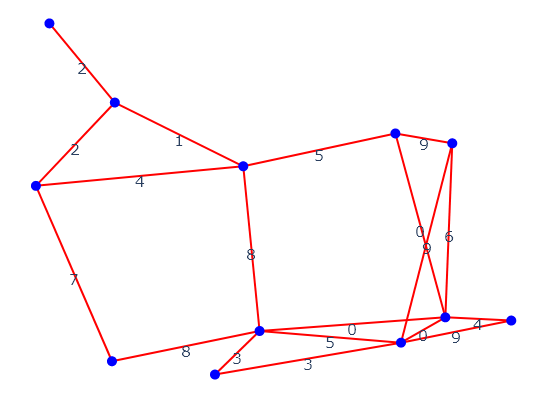A weighted graph

The procedure can be explained as follows: we let a parameter $$\varepsilon$$ start at 0, and as we increase it all the way to infinity we keep considering the instantaneous subgraphs made of a) all the vertices in the original graph, and b) those edges whose weight is less than or equal to the current $$\varepsilon$$. We also promote these subgraphs to more general structures called (simplicial) complexes that, alongside vertices and edges, also possess $$k$$-simplices, i.e. selected subsets of $$k + 1$$ vertices (a 2-simplex is an abstract “triangle”, a 3-simplex an abstract “tetrahedron”, etc). Our criterion is this: for each integer $$k \geq 2$$, all $$(k + 1)$$-cliques in each instantaneous subgraph are declared to be the $$k$$-simplices of the subgraph’s associated complex. By definition, the $$0$$-simplices are the vertices and the $$1$$-simplices are the available edges.

As $$\varepsilon$$ increases from 0 (included) to infinity, we record the following information:

1. How many new connected components are created because of the appearance of vertices (in this example, all vertices “appear” in one go at $$\varepsilon = 0$$, by definition!), or merge because of the appearance of new edges.

2. How many new 1-dimensional “holes”, 2-dimensional “cavities”, or more generally $$d$$-dimensional voids are created in the instantaneous complex. A hole, cavity, or $$d$$-dimensional void is such only if there is no collection of “triangles”, “tetrahedra”, or $$(d + 1)$$-simplices which the void is the “boundary” of. Note: Although the edges of a triangle alone “surround a hole”, these cannot occur in our particular construction because the “filling” triangle is also declared present in the complex when all its edges are.

3. How many $$d$$-dimensional voids which were present at earlier values of $$\epsilon$$ are “filled” by $$(d + 1)$$-simplices which just appear.

This process of recording the full topological history of the graph across all edge weights is called (Vietoris-Rips) persistent homology.

Let us start at $$\varepsilon = 0$$: Some edges had zero weight in our graph, so they already appear!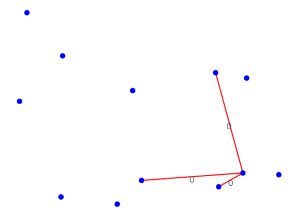$$\varepsilon = 0$$

There are 9 connected components, and nothing much else.

Up to and including $$\varepsilon = 2$$, a few more edges are added which make some of the connected components merge together but do not create any hole, triangles, or higher cliques. Let us look at $$\varepsilon = 3$$: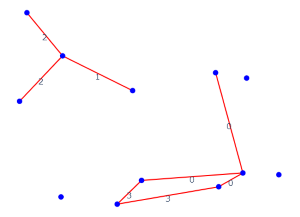$$\varepsilon = 3$$

The newly arrived edges reduce the number of connected components further, but more interestingly they create a 1D hole!

As an example of a “higher”-simplex, at $$\varepsilon = 4$$ we get our first triangle: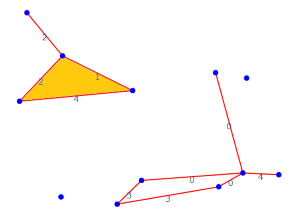$$\varepsilon = 4$$

At $$\varepsilon = 5$$, our 1D hole is filled: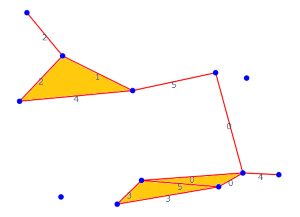$$\varepsilon = 5$$

At $$\varepsilon = 8$$, two new 1D holes appear: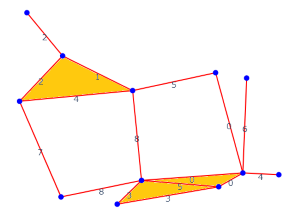$$\varepsilon = 8$$

Finally, at $$\varepsilon = 9$$, some more connected components merge, but no new voids are either created or destroyed: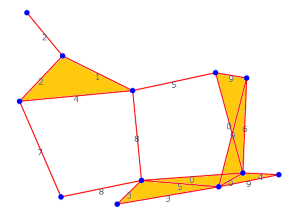$$\varepsilon = 9$$

We can stop as we have reached the maximum value of $$\varepsilon$$, beyond which nothing will change: there is only one connected component left, but there are also two 1D holes which will never get filled.

Fit-transforming via VietorisRipsPersistence(metric="precomputed") on the original graph’s adjacency matrix would return the following 3D array of persistence diagrams:

diagrams = np.array([[[0., 1., 0],
[0., 2., 0],
[0., 2., 0],
[0., 3., 0],
[0., 4., 0],
[0., 5., 0],
[0., 6., 0],
[0., 7., 0],
[3., 5., 1],
[8., np.inf, 1],
[8., np.inf, 1]]])


The notebook Topological feature extraction using VietorisRipsPersistence and PersistenceEntropy explains how to interpret this output and how to make informative 2D scatter plots out of its entries. Here, we only have one entry corresponding to our graph:

from gtda.plotting import plot_diagram

plot_diagram(diagrams)
`